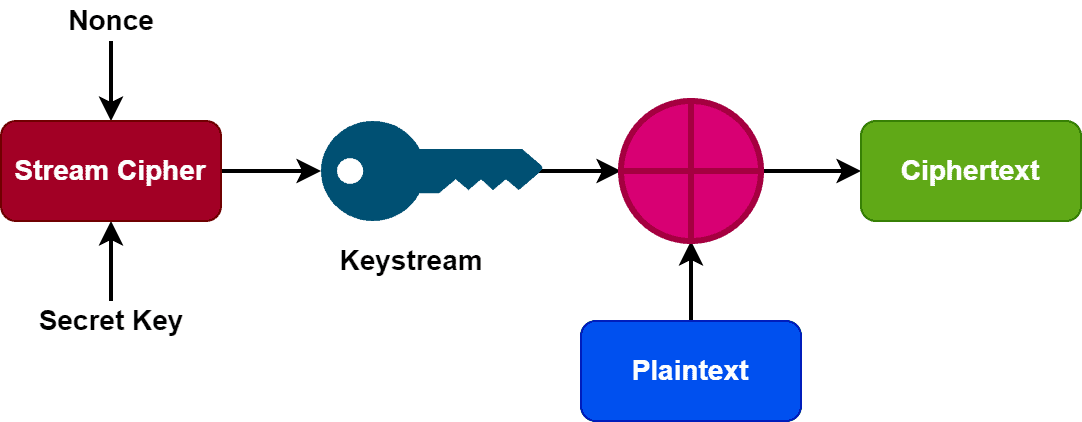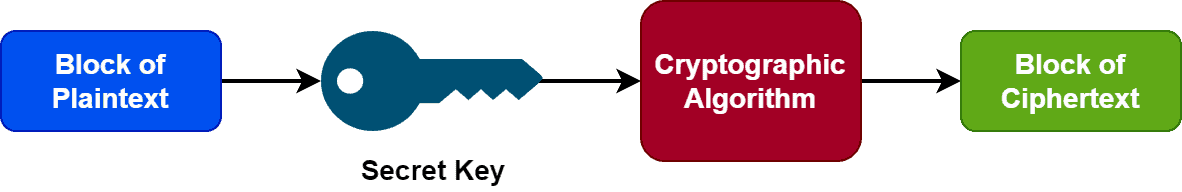## 1. Overview

In this tutorial, we’ll discuss two cryptographic encryption techniques: stream and block ciphers. We’ll present the working procedure of both methods with examples.

Finally, we’ll discuss the core differences between them.

## 2. Introduction to Ciphers

Cryptography generally protects a system from cyber attackers by securing communication between two systems. A cryptographic process starts with a given plaintext. We generate an encrypted ciphertext from the given plaintext using an encryption algorithm. In order to get back the original plaintext message, we utilize a decryption algorithm:The encryption and decryption algorithms are known as ciphers in cryptography. Ciphers use keys in order to encrypt and decrypt messages. Based on the usage of the key, we can divide ciphers into two broad categories: symmetric and asymmetric.

A symmetric cipher utilizes a shared key for the encryption and decryption process. Because of the usage of a single key, symmetric ciphers are fast and can be applied when the amount of data is large. However, it can only facilitate the confidentiality of the data. Additionally, the key used for the whole process is secret and only shared between sender and receiver:On the other hand, an asymmetric cipher utilizes different keys for the encryption and decryption process. Due to the usage of different keys, it takes more time compared to a symmetric cipher. Therefore, an asymmetric cipher is a good choice when the available data is small. The significant advantage is preserving confidentiality along with authenticity and non-repudiation of the data. Additionally, we can share the key used for the encryption process with everyone. However, the key to the decryption process is secret:## 3. Stream Cipher

Stream cipher comes under the category of the symmetric cipher. It encrypts a given plain text to a cipher text using a secret key.

It utilizes a key (128/256 bits) and a nonce digit (64-128 bits) to convert the plaintext to ciphertext. Both the key and nonce digit creates a keystream of pseudorandom bits. Additionally, it uses time-varying transformations on the given plaintext and works bit-by-bit basics.

Finally, we perform the XOR operation between each bit of keystream and plaintext in order to generate the ciphertext:We repeat this whole process for all the bits of the plaintext. Additionally, it’s essential not to use the same key and nonce combination throughout the process. Using the same combination can result in a duplicate keystream.

We can further divide stream ciphers into two categories: synchronous and self-synchronizing stream ciphers. In a synchronous stream cipher, the generation of the keystream block is neither dependent on the plaintext nor the ciphertext. However, the generation of the keystream block of an asynchronous stream cipher depends on the previous ciphertext.

Examples of stream cipher include ChaCha20, Salsa20, A5/1, and RC4.

Let’s take an example. Let’s assume the first alphabet of the plaintext is D. The binary equivalent of the letter D based on the ASCII table is 01000100. Additionally, the given keystream for encryption and decryption is 11001100. Now in order to produce the ciphertext, we need to perform an XOR operation between plaintext and keystream. Hence, the ciphertext for this particular example will be 10001000. We can regenerate the original plaintext by performing an XOR operation between ciphertext and keystream.

## 4. Block Cipher

Like a stream cipher, a block cipher encrypts a block of given plaintext using a key and a cryptographic algorithm. In contrast to a stream cipher, a block cipher takes fixed-size blocks of plaintext and produces fixed-size blocks of ciphertext. Generally, the size of the plaintext blocks is either 64 or 128 bits:A block cipher utilizes a symmetric algorithm and key during the encryption-decryption process. Additionally, it uses an initialization vector generated with a random number generator. The initialization vector and the symmetric secret key are added in the first plaintext block. Adding the initialization vector ensures we can’t regenerate the secret key with a brute force process. Additionally, it provides no duplication among the subsequent ciphertext blocks.

Now let’s discuss some modes of operation in the block cipher.

Each mode in block cipher defers by the way encryption is defined. Electronic codebook (ECB) is the simplest of all block cipher modes. It electronically codes messages and works without randomly generating the secret keys. ECB works on a single-bit stream. Additionally, it encrypts each plaintext block independently.

Another popular mode is cipher block chaining (CBC). It combines each block of plaintext with the previous block in order to perform the encryption process. The block of ciphertext generated by CBC depends on all the earlier blocks of plaintext in a data stream. In the OSI model, the transport layer uses CBC to encrypt data.

A block cipher’s efficiency depends on the block’s size and secret key. The randomness in the key is essential. Additionally, the block size shouldn’t be either very small or large. In general, the block size should be multiple of 8 bits. Some examples of block cipher are data encryption standard (DES), triple DES (3DES or TDEA), and advanced encryption standard (AES).

## 5. Differences

Let’s discuss some core differences between stream and block ciphers:## 6. Conclusion

In this tutorial, we discussed stream and block ciphers in detail. We also presented the core differences between them.